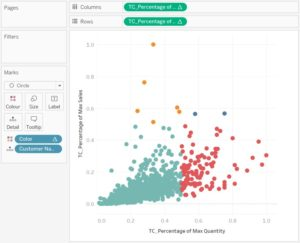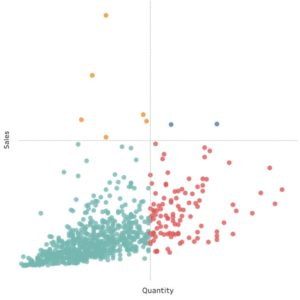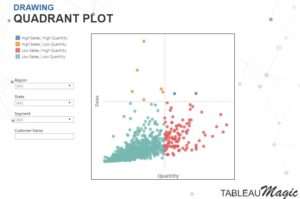# PATH Tips/Best Practices- How to creat a quadrant chart

Posted in dicas tableau

In this tip you will learn how to create quadrant charts that help you segment your data quickly. For this we will use the order database available in Tableau.Step 1:   In a new worksheet, Create the following Calculated Fields:

• TC_Percentage of Max Quantity:

SUM([Quantity])/WINDOW_MAX(SUM([Quantity]))

• TC_Percentage of Max Sales:

SUM([Sales])/WINDOW_MAX(SUM([Sales]))

• Color:

IF [TC_Percentage of Max Sales] <0.5 AND [TC_Percentage of Max Quantity] < 0.5 THEN

“Low Sales / Low Quantity”

ELSEIF [TC_Percentage of Max Sales] >= 0.5 AND [TC_Percentage of Max Quantity] < 0.5 THEN

“High Sales / Low Quantity”

ELSEIF [TC_Percentage of Max Sales] >= 0.5 AND [TC_Percentage of Max Quantity] >= 0.5 THEN

“High Sales / High Quantity”

ELSE

“Low Sales / High Quantity”

END

Step 2: On the tag card, change the chart type to circles. Drag the customer name to detail tag. After that, drag the calculated field TC_Postage Percentage to columns and TC_Postage Maximum Percentage to rows. Add the third calculated field named Color to the Color tag card. Finally, right-click this object, go to Compute Using, and select Client Name.Step 3: Add a trendline to the X axis, create a parameter, and set the value to 0.5. After this, add a trendline to the Y axis. Hide the major tick marks, the grid line, and the zero lines: